This example uses the World Indicators saved data source, which comes with Tableau. We’ll use the MODEL_QUANTILE and MODEL_PERCENTILE predictive modelling functions to explore the relationships between health spending per capita, female life expectancy, birth rate.

Let’s start with a visualisation that compares each country’s health spending with its female life expectancy. To follow along and access the pre-built views and dashboards, or to view the solution, download the following workbook from Tableau Public: Predictive Modelling of Female Life Expectancy.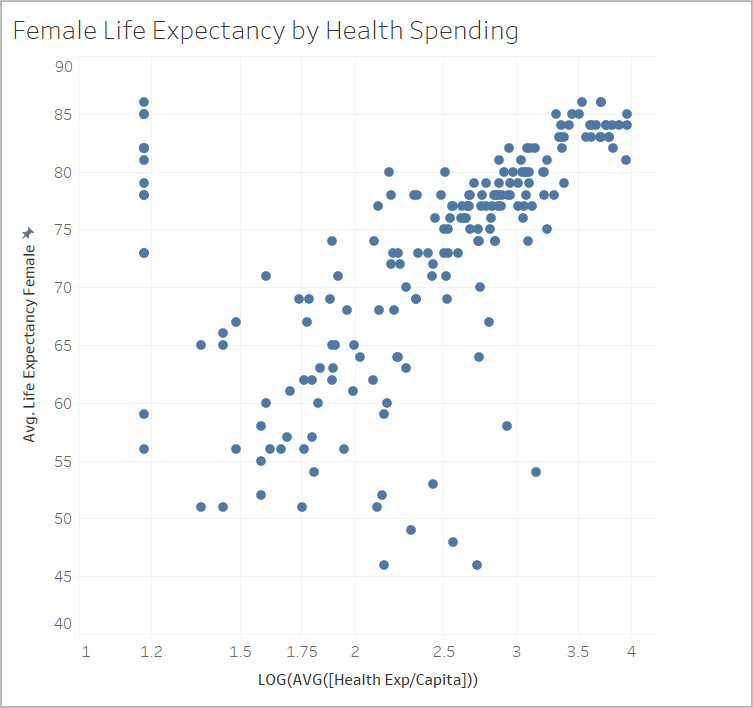## Using MODEL_PERCENTILE

First we’ll evaluate the life expectancy and health expenditures for all visible marks. This will allow Tableau to build a model from those marks and return the percentile for each within the model.

### Step 1: Create the prediction calculation

If you also have Tableau Server or Tableau Cloud and you want to do your authoring on the web instead of in Tableau Desktop, publish the workbook to your Tableau server, click Workbooks, select the workbook, then under Actions, choose Edit Workbook.

Once you open the workbook, you'll see that it has several sheets. You'll be using those sheets to build your views.

1. In the starter workbook, click the Percentile Starter sheet.

2. Open the Analysis menu at the top, and then select Create Calculated Field.

3. In the Calculation Editor, do the following:

• Name the calculation: Percentile Expectancy vs Spending

• Note: If you’re following along in the starter workbook, you’ll see different names for the calculations used throughout this example. This is so that you’ll be able to name the fields as described here, without creating duplicates. Your solution may have different names, but the visualisations should look the same.
• Enter the following formula:

`MODEL_PERCENTILE(AVG([Life Expectancy Female]), LOG(MEDIAN([Health Exp/Capita])))`

• This calculation uses average life expectancy as the target expression, and median health expenditure as the predictor. In this case, we used a logarithmic transformation on the health spending axis, as well as for the predictor.

Note: Because this data set skews toward very large values, we transformed our data using a logarithmic scale, which is useful when analysing data with a few values that are much higher than the rest, and will make it easier to identify trends and relationships in our data set.
4. Click OK.

The prediction calculation is now added as a calculated field in the Data pane.

### Step 2: Add the prediction calculation to the view

In the viz above, you can see each country's health spending against its female life expectancy, filtered to 2012.

Now, let’s add the MODEL_PERCENTILE calculation to the view and see what insights we can gain.

1. Drag Percentile Expectancy vs Spending to Colour on the Marks card.

2. Click the drop-down arrow on the pill and select Compute Using > Country/Region.

3. Click Colour on the Marks card, and then click Edit Colours.

• Under Palette, select Orange-Blue Diverging.

• Select the Stepped Colour tick box.

• Select the Reversed tick box.

4. Click OK.

5.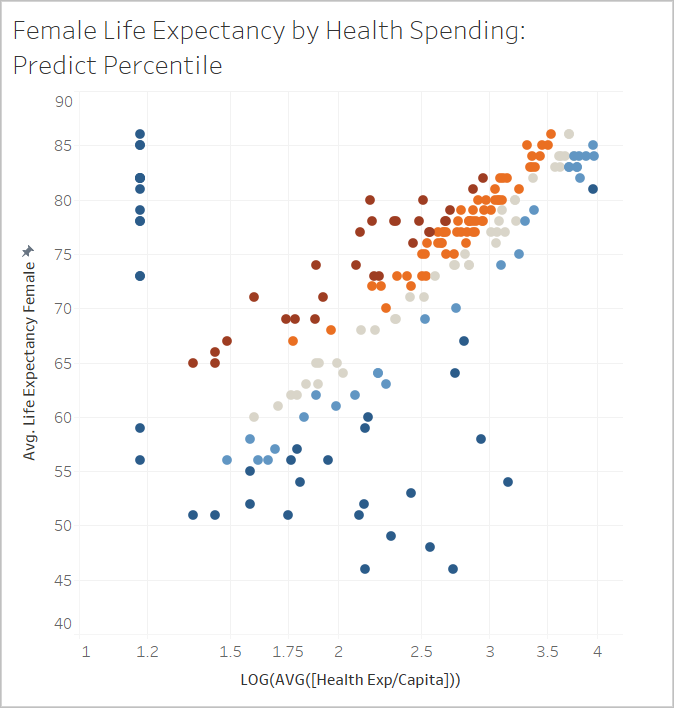You can see the distribution of countries where health expectancy is both higher and lower than expected based on the level of spending. Notice that generally, the dark red marks indicate that life expectancy is high relative to healthcare spending, dark blue means that life expectancy is low relative to healthcare spending, and grey means that life expectancy is close to what the model expects, based on the level of healthcare spending.

### Step 3: Group the results by colour

To simplify analysis, let’s use the prediction calculation within a new calculation to group the results. We’ll build groups so that marks above the 90th percentile and below the 10th percentile are grouped together, marks in the 80th–90th percentile range and 10th–20th percentile range are grouped together, and so on. We’ll also highlight marks with a null value and address those later using the other predictive modelling function, MODEL_QUANTILE.

1. In the Calculation Editor, do the following:

• Name the calculation: Percentile by Colour.

• Enter the following formula:

```IF ISNULL([Percentile Expectancy vs Spending]) THEN "Null" ELSEIF [Percentile Expectancy vs Spending] >=0.9 OR [Percentile Expectancy vs Spending] <=0.1 THEN "<10th & >90th percentile" ELSEIF [Percentile Expectancy vs Spending] >=0.8 OR [Percentile Expectancy vs Spending] <=0.2 THEN "<20th & >80th percentile" ELSEIF [Percentile Expectancy vs Spending] >=0.7 OR [Percentile Expectancy vs Spending] <=0.3 THEN "<30th & >70th percentile" ELSEIF [Percentile Expectancy vs Spending] >=0.6 OR [Percentile Expectancy vs Spending] <=0.4 THEN "<40th & >60th percentile" ELSE "50th percentile +-10" END```

2. Add the new calculation to Colour on the Marks card.

3. Click the drop-down arrow on the pill and select Compute Using > Country/Region.

4. Click Colour on the Marks card, and then click Edit Colours.

• Adjust the colours to better see the trend. In this case, let’s use the Traffic Light colour palette, and use grey for Nulls.

5. Click OK.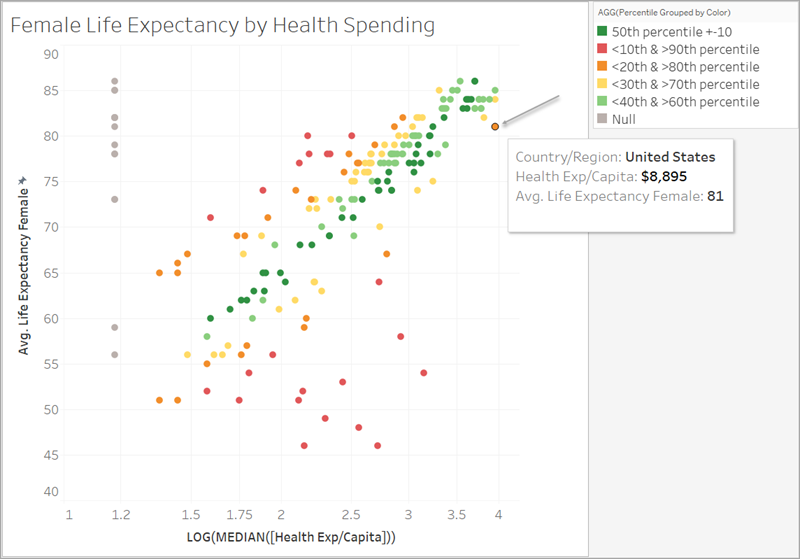Looking at the orange mark in the corner, notice that the US spends \$8,895 per female for a life expectancy of 81 years. Moving along the X-axis to the left, you can see that other countries spend less and have the same life expectancy.

The model evaluates the strength of the relationship at each point, where the US is close to the upper end of the model’s expected range.

### Step 4: Compare life expectancy with birth rate

Next, let’s look at a viz that compares female life expectancy with birth rate. Notice that there is a negative correlation between birth rates and female life expectancy; however, this does not mean that higher birth rates cause lower female life expectancy. There are likely additional factors that affect both birth rates and female life expectancy that are not visible in this view of the data. But let’s add the model and see where the model expects female life expectancy to be higher or lower given health expenditures.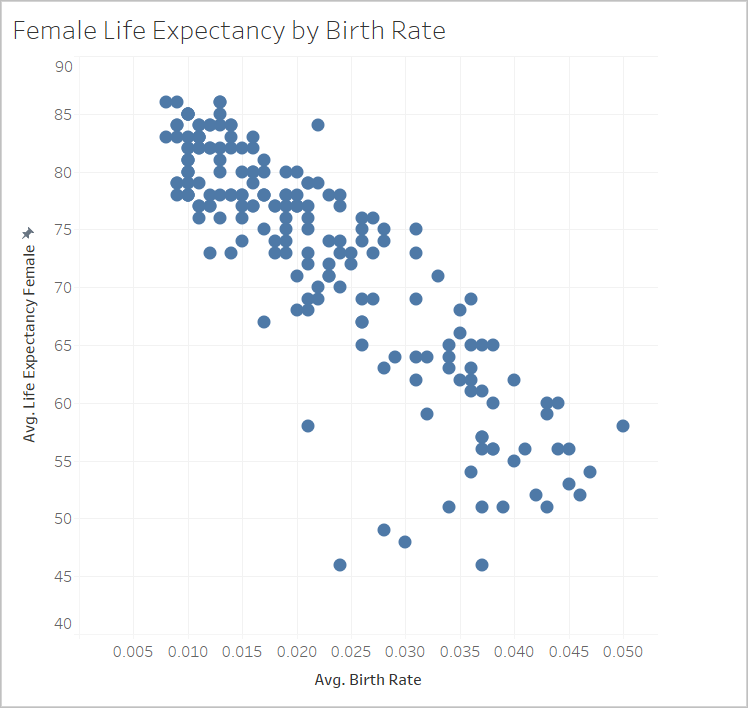1. On the Birth Rate sheet, add the Percentile by Colour prediction calculation to Colour on the Marks card to bring it into the view.

2. Click the drop-down arrow on the pill and select Compute Using > Country/Region.

3. Click Colour on the Marks card, and click Edit Colours. Edit the colours as before, using the Traffic Light palette and grey for Null.

4. Click OK.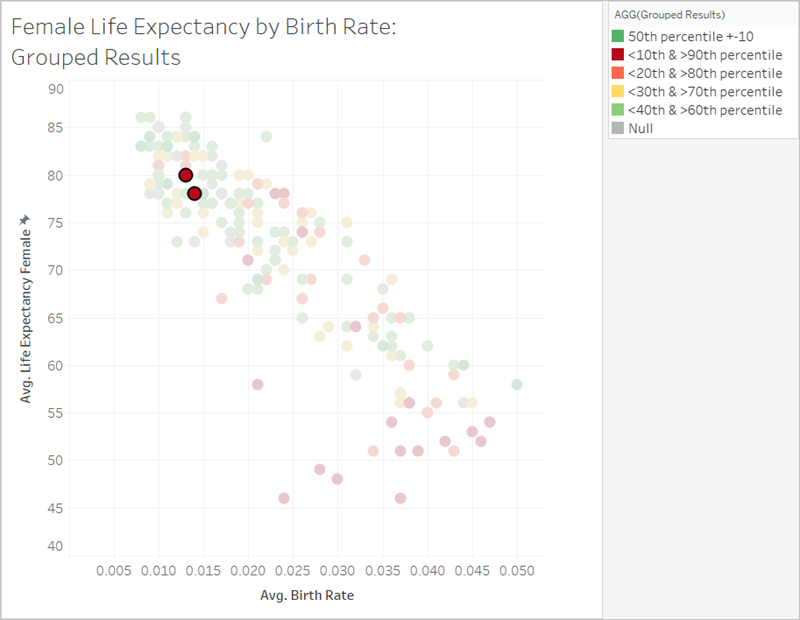Now the data is much more distributed. The red band in the lower right corner is where life expectancy is lowest but the birth rate is highest, and healthcare spending relative to life expectancy is low. Singling out the two red marks in the top left quadrant, which pertain to Albania and Armenia, you’ll notice that both countries have high female life expectancy, lower birth rates and low health expenditures.

As you can see, we were able to use MODEL_PERCENTILE to identify that these two countries are outliers: Even though they both had relatively low healthcare spending, they still have relatively high life expectancies, placed in the context of birth rate.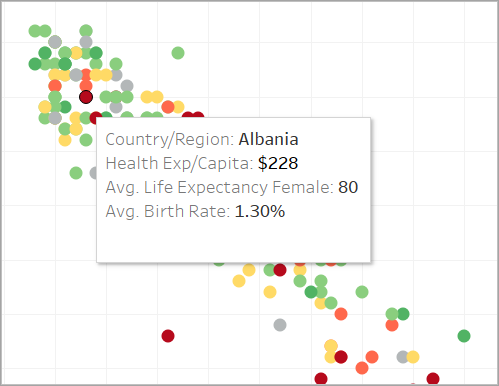5. Now, let’s see how you can use the other predictive modelling function, MODEL_QUANTILE, to continue your analysis.

## Using MODEL_QUANTILE

MODEL_QUANTILE is used to generate numeric predictions, given a target percentile, target expression and predictors. It’s the inverse of MODEL_PERCENTILE.

Recall that we have several null values in our results, meaning that some countries don’t have health expenditure data. We’ll use MODEL_QUANTILE to estimate those missing values.

### Step 1: Create the prediction calculations

We’ve been working with this calculation:

`MODEL_PERCENTILE(AVG([Life Expectancy Female]), LOG(MEDIAN([Health Exp/Capita])))`

First, we want to invert this function in order to get a prediction for healthcare expenditure based on female life expectancy.

1. In the starter workbook, click the Quantile Starter sheet.

2. Open the Analysis menu at the top, and then select Create Calculated Field.

3. In the Calculation Editor, do the following:

• Name the calculation: Quantile of Life Expectancy vs Spending
• Enter the following formula:

```POWER(10, MODEL_QUANTILE(0.5, LOG(MEDIAN([Health Exp/Capita])), AVG([Life Expectancy Female]))) ```

Let’s break this calculation down to better understand it:

• We start with MODEL_QUANTILE, where the first argument is 0.5, specifying what percentile to predict.
• The target expression is median health expenditure per capita.
• The predictor is average female life expectancy.
• In addition, we wrapped the function inside a POWER function to convert the log-transformed target expression back into dollars.
4. Click OK.

The prediction calculation is now added as a calculated field in the Data pane.

### Step 2: Add the prediction calculation to the view

1. Drag Quantile of Life Expectancy vs Spending to Tooltip on the Marks card.

2. Click the drop-down arrow on the pill and select Compute Using > Country/Region.

3. Click Tooltip on the Marks card and add a row for the MODEL_QUANTILE prediction:

• Name the tooltip row: Predicted Health Spend from Female Life Expectancy:

• Click Insert and select the calculation to ensure the tooltip will dynamically show the mark’s unique prediction as you interact with the viz.

4. Click OK.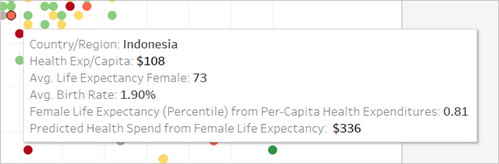5. Right now our MODEL_QUANTILE calculation only has one predictor: female life expectancy. Notice as you move from left to right, viewing the tooltip for marks with the same life expectancy, that each one has the same predicted health spend. Indonesia’s predicted health spend from life expectancy is \$336, but so is Fiji’s, Egypt’s and other countries that have the same female life expectancy.

This is because the model provides the same estimated expenditure for each mark. Since we're only using one predictor (female life expectancy), the model returns the same results for all marks where the predictor has the same value. You can add more nuance to the model by adding predictors.

### Step 3: Add a prediction with a second predictor

As you build your predictions, you should consider which fields will be good predictors for your target values and include them in your calculations. You can combine any number of dimensions and measures. For example, we could add GDP, population and other fields as predictors to improve our predictions. In this case, let’s add Region.

1. In the Calculation Editor, do the following:

• Name the calculation: Quantile Spend vs Expectancy & Region

• Enter the following formula, which is the same as the previous calculation, but adds Region as a predictor:

`POWER(10, MODEL_QUANTILE(0.5, LOG(MEDIAN([Health Exp/Capita])), AVG([Life Expectancy Female]), ATTR([Region])))`

2. Click OK.

3. Next, add the new calculation to Tooltip on the Marks card.

4. Click Tooltip, and add another line to describe the new prediction, such as Predicted Health Spend from Female Life Expectancy & Region:.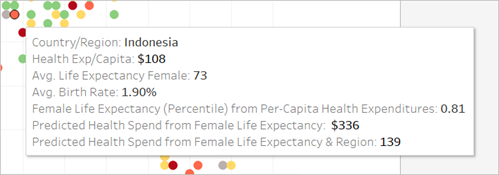Now the tooltip shows both predictions.

### Step 4: Compare actual values to predicted values

As a final step in your analysis, you can also build prediction calculations that combine actual and predicted values. In our example, let’s show the actual health expenditures where available and the estimated expenditures where unavailable.

1. In the Calculation Editor, do the following:

• Name the calculation: Health Spend Actual vs Prediction Value

• Enter the following formula, which will return the numeric value of the prediction:

```ROUND(IFNULL(AVG([Health Exp/Capita]),[Quantile_HE/Cap_LEF,Region]),0) ```

2. Click OK.

3. Create another calculation as follows:

• Name the calculation: Health Spend Actual vs Prediction Tag

• Enter the following formula, which will act as a label for the above calculation:

```STR(IF ISNULL(AVG([Health Exp/Capita])) THEN "(Estimate)" ELSE "(Actual)" END) ```

4. Click OK.

5. Next, add both calculations to Tooltip on the Marks card.

6. Click Tooltip, and add another line to describe the new calculations:

• Per Capita Health Expenditure (Actual or Estimated):

• Insert the new calculations, one after the other.

7. Click OK.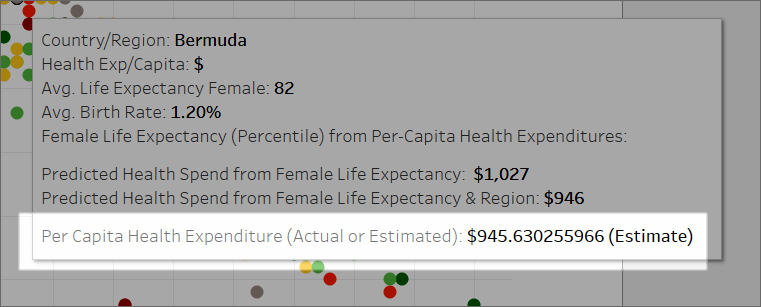Now, as you interact with the viz, you can see each country’s per capita health spending, or view an estimate if the actual value was missing (null) from the data.

Just like that, you can use predictive modelling functions in Tableau to gain insights into your data.